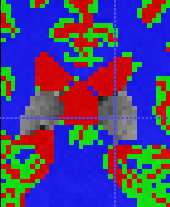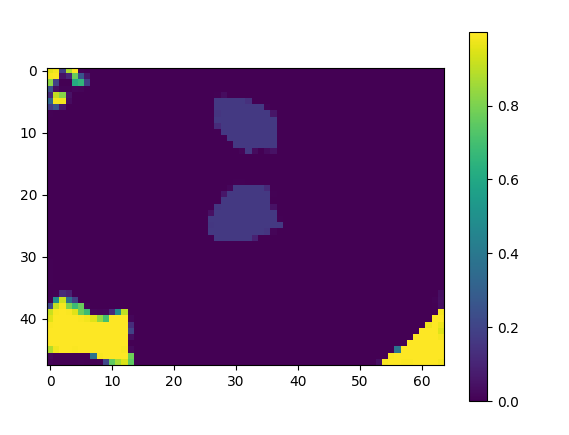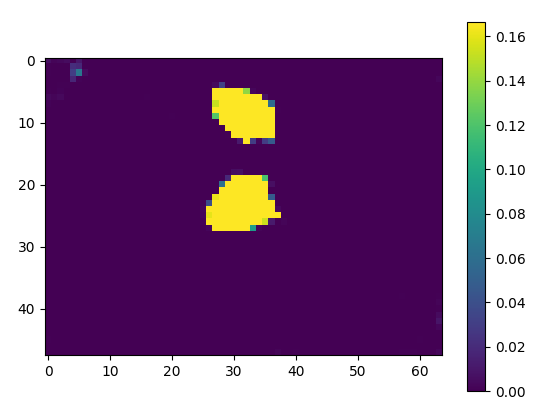# Getting proper class predictions in multi-class segmentation

Hello,
I am using the following codes to finding the class probabilities to classify channels into different labels. The training was of 6 class labels`[0,1,2,3,4,56]` in a one-hot encoded method.

``````for bIdx, sample in enumerate(test_loader):
img, seg = sample.to(device), sample.to(device)   # shape --> [2,6,48,64,64]
pred = model(img)
lbl_pred = pred.data.max(1).cpu().numpy()[:, :, :].astype('uint8')
``````

But I am missing `label 5` in the prediction/output(the cursor in the following image).This shows as `label 0` which is clearly not the case as label 0: background.
But if I check `pred.data` separately, it has label 5 probability values in it.

``````plt.imshow(pred.data[0, 5, :, 20, :].cpu().numpy())
plt.show()
``````

resulting in the following image.Am I missing anything?

While the class5 channel would have probabilities (or logits) for this class, the max. logit for these pixels could still be class0. Did you compare the values of both classes for the area of interest?

1 Like

This is for channel 0:

``````c = plt.imshow(pred.data[0, 0, :, 20, :].cpu().numpy())
``````This is for channel 5:

``````c = plt.imshow(pred.data[0, 5, :, 20, :].cpu().numpy())
````````````c = plt.imshow(pred.data[0, 1, :, 20, :].cpu().numpy())
``````

Channel 1:I’m not the best at color matching, but I guess that the “yellow” output for channel5 is at `~0.16`, while the output for channel0 would be at `~0.2`. To better compare these outputs you could use the same scale for the probabilities (use `[0, 1]` as it would be the limit) or compare the values directly.

I guess the value in channels 0 and 5 are the same for the anatomy.

Channel 5:Channel 0:I think it is because the tissue didn’t train well as visible from the dice score(TP(valid/black)).

How can you show all the channels?